# DHT11 Humidity & Temperature Sensor Module

This module integrates DHT11 sensor and other required components on a small PCB.  The DHT11 sensor includes a resistive-type humidity measurement component, an NTC temperature measurement component and a high-performance 8-bit microcontroller inside, and provides calibrated digital signal output.  It has high reliability and excellent long-term stability, thanks to the exclusive digital signal acquisition technique and temperature & humidity sensing technology.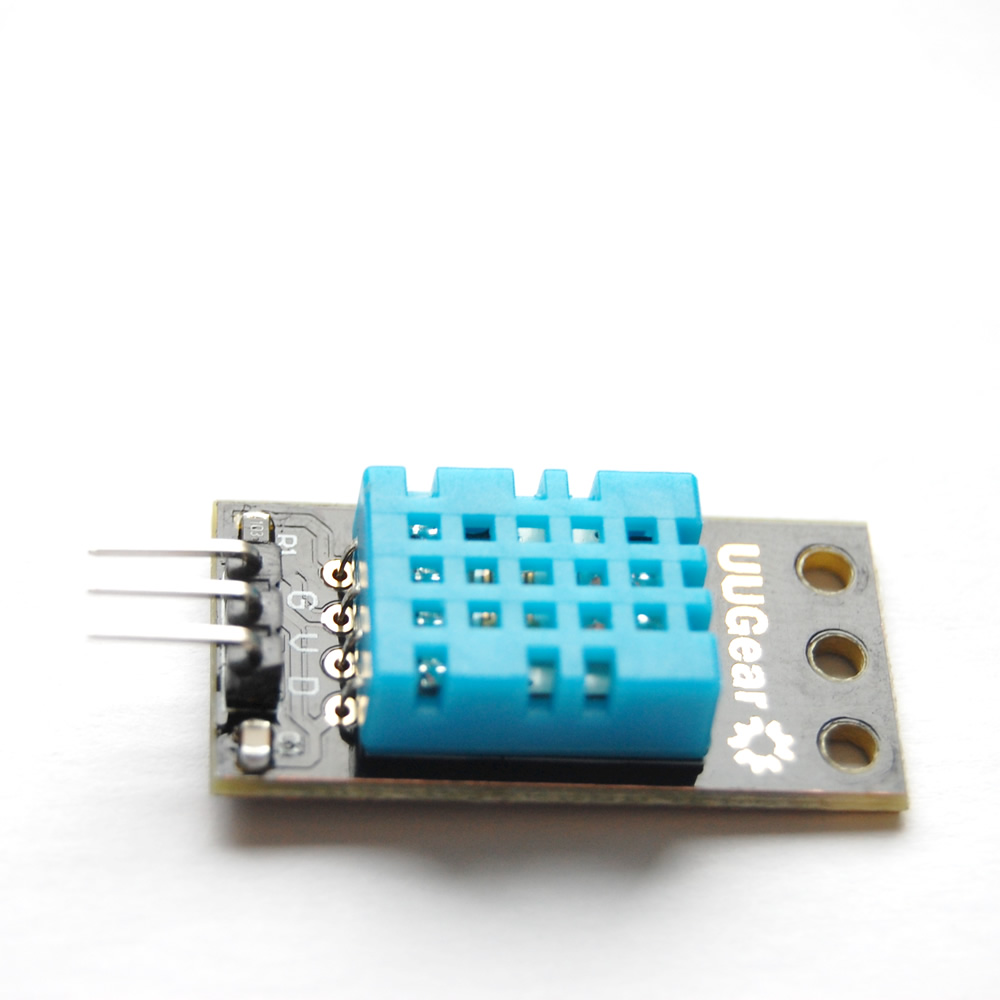Each DHT11 is strictly calibrated in the laboratory that is extremely accurate on humidity calibration. The calibration coefficients are stored as programmes in the OTP memory, which are used by the sensor’s internal signal detecting process. The single-wire serial interface makes system integration quick and easy. Its small size, low power consumption and up-to-20 meter signal transmission making it the best choice for various applications, including those most demanding ones. The component is 4-pin single row pin package. It is convenient to connect and special packages can be provided according to users’ request.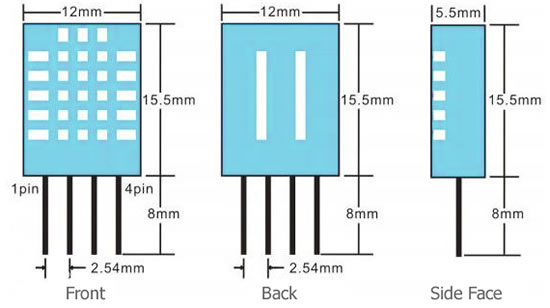The module is actually a PCB that has DHT11 component soldered with a few components, and it is a 3-wire module: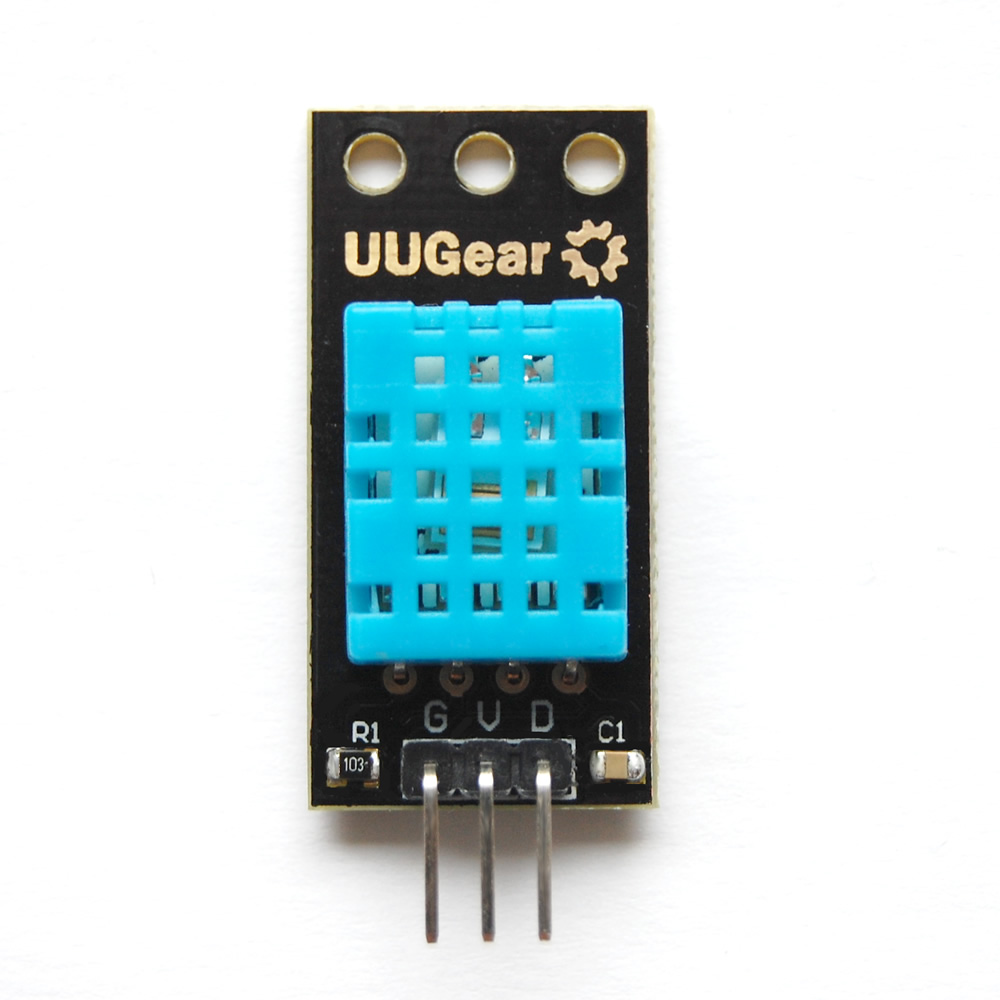1.    VCC connected to +3.3V~5V
2.    DATA connected to the microcontroller IO port
3.    GND connected to ground

### Technical Specifications：

Power Supply： 3.3~5.5V DC
Output： 4 pin single row
Measurement Range： Humidity 20-90%RH， Temperature  0~50℃
Accuracy： Humidity +-5%RH， Temperature +-2℃
Resolution： Humidity  1%RH， Temperature  1℃
Interchangeability： Fully Interchangeable
Long-Term Stability： <±1%RH/year

## How to Process the Data

Single bus data format is used for the communication and synchronization between MCU and the DHT11 sensor. Each communication process will last about 4ms.
The data is transmitted in this format:

• 8bit integral RH data +
• 8bit decimal RH data +
• 8bit integral T data +
• 8bit decimal T data +
• 8bit check sum.

If the data transmission is correct, the check sum should equals to the lower 8bit of the result of “8bit integral RH data + 8bit decimal RH data + 8bit integral T data + 8bit decimal T data”.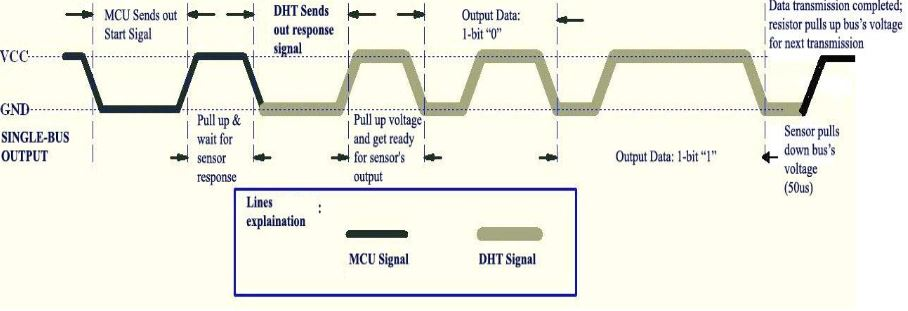The default status of the DATA pin is high. When the communication between MCU and DHT11 starts, MCU will pull down the DATA pin for least 18ms. This is called “Start Signal” and it is to ensure DHT11 has detected the signal from MCU.  Then MCU will pull up DATA pin for 20-40us to wait for DHT11’s response.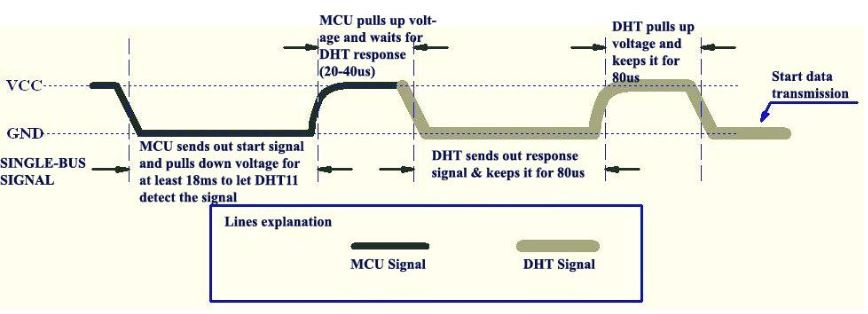Once DHT11 detects the start signal, it will pull down the DATA pin as “Response Signal”, which will last 80us. Then DHT11 will pull up the DATA pin for 80us, and prepare for data sending.
During the data transition, every bit of data begins with the 50us low-voltage-level and ends with a high-voltage-level signal. The length of the high-voltage-level signal decides whether the bit is “0” or “1”.
Data bit “0” has 26-28us high-voltage length: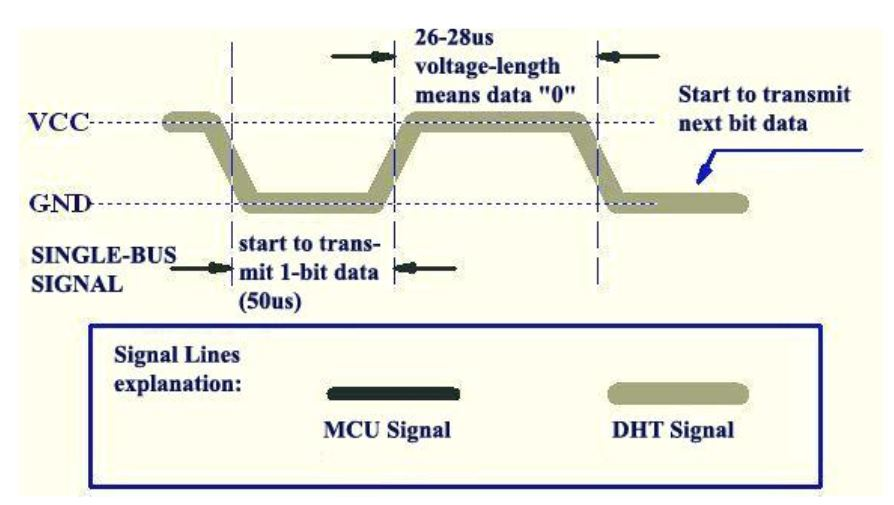While data bit “1” has 70us high-voltage length:## Connection with GPIOs on Raspberry Pi

Besides the VCC and GND, you just need to use one GPIO pin on the Raspberry Pi to make use of the DHT11 module.
Raspberry Pi                   DHT11 Module
3.3v P1  —————————–  VCC (V)
GND P6  —————————-  GND (G)
GPIO4 P7 —————————–  DATA (S)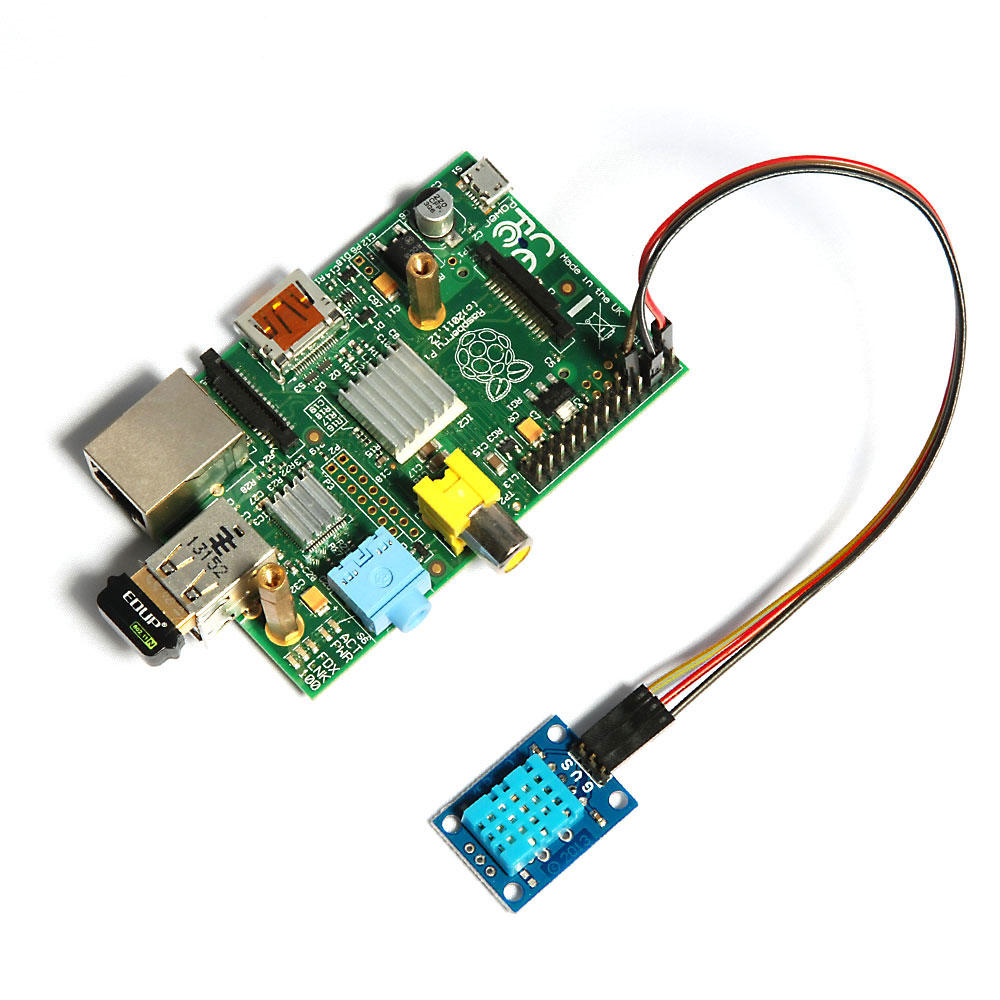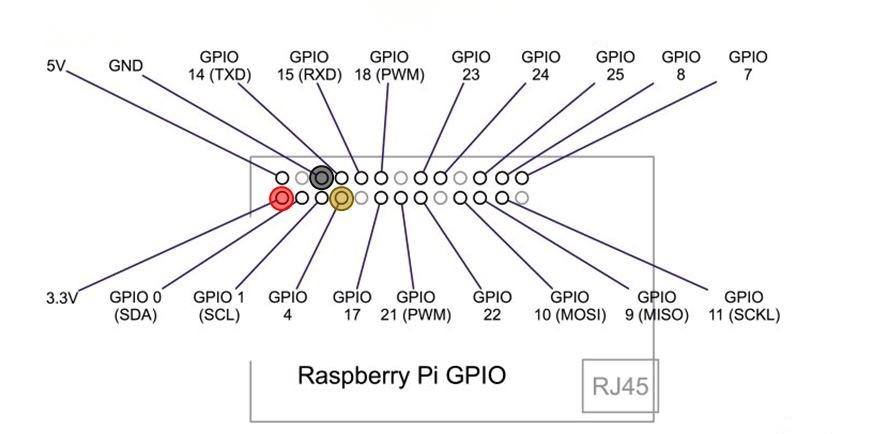## Code Examples

Here is an example written in C:

```/*
*  dht11.c:
*	Simple test program to test the wiringPi functions
*	DHT11 test
*/
#include <wiringPi.h>
#include <stdio.h>
#include <stdlib.h>
#include <stdint.h>
#define MAXTIMINGS	85
#define DHTPIN		7
int dht11_dat = { 0, 0, 0, 0, 0 };
{
uint8_t laststate	= HIGH;
uint8_t counter		= 0;
uint8_t j		= 0, i;
float	f; /* fahrenheit */
dht11_dat = dht11_dat = dht11_dat = dht11_dat = dht11_dat = 0;
/* pull pin down for 18 milliseconds */
pinMode( DHTPIN, OUTPUT );
digitalWrite( DHTPIN, LOW );
delay( 18 );
/* then pull it up for 40 microseconds */
digitalWrite( DHTPIN, HIGH );
delayMicroseconds( 40 );
/* prepare to read the pin */
pinMode( DHTPIN, INPUT );
/* detect change and read data */
for ( i = 0; i < MAXTIMINGS; i++ )
{
counter = 0;
while ( digitalRead( DHTPIN ) == laststate )
{
counter++;
delayMicroseconds( 1 );
if ( counter == 255 )
{
break;
}
}
if ( counter == 255 )
break;
/* ignore first 3 transitions */
if ( (i >= 4) && (i % 2 == 0) )
{
/* shove each bit into the storage bytes */
dht11_dat[j / 8] <<= 1;
if ( counter > 16 )
dht11_dat[j / 8] |= 1;
j++;
}
}
/*
* check we read 40 bits (8bit x 5 ) + verify checksum in the last byte
* print it out if data is good
*/
if ( (j >= 40) &&
(dht11_dat == ( (dht11_dat + dht11_dat + dht11_dat + dht11_dat) & 0xFF) ) )
{
f = dht11_dat * 9. / 5. + 32;
printf( "Humidity = %d.%d %% Temperature = %d.%d *C (%.1f *F)\n",
dht11_dat, dht11_dat, dht11_dat, dht11_dat, f );
}else  {
printf( "Data not good, skip\n" );
}
}
int main( void )
{
printf( "Raspberry Pi wiringPi DHT11 Temperature test program\n" );
if ( wiringPiSetup() == -1 )
exit( 1 );
while ( 1 )
{
delay( 1000 ); /* wait 1sec to refresh */
}
return(0);
}```

Here is a screen shot for this example: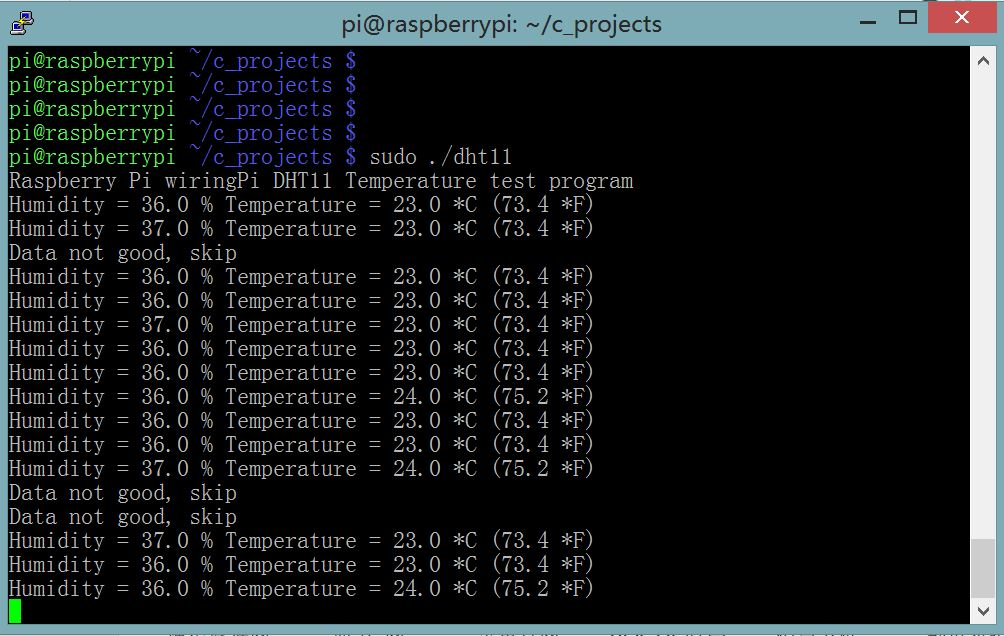Here is another example written in Python:

```import RPi.GPIO as GPIO
import time
def bin2dec(string_num):
return str(int(string_num, 2))
data = []
GPIO.setmode(GPIO.BCM)
GPIO.setup(4,GPIO.OUT)
GPIO.output(4,GPIO.HIGH)
time.sleep(0.025)
GPIO.output(4,GPIO.LOW)
time.sleep(0.02)
GPIO.setup(4, GPIO.IN, pull_up_down=GPIO.PUD_UP)
for i in range(0,500):
data.append(GPIO.input(4))
bit_count = 0
tmp = 0
count = 0
HumidityBit = ""
TemperatureBit = ""
crc = ""
try:
while data[count] == 1:
tmp = 1
count = count + 1
for i in range(0, 32):
bit_count = 0
while data[count] == 0:
tmp = 1
count = count + 1
while data[count] == 1:
bit_count = bit_count + 1
count = count + 1
if bit_count > 3:
if i>=0 and i<8:
HumidityBit = HumidityBit + "1"
if i>=16 and i<24:
TemperatureBit = TemperatureBit + "1"
else:
if i>=0 and i<8:
HumidityBit = HumidityBit + "0"
if i>=16 and i<24:
TemperatureBit = TemperatureBit + "0"
except:
print "ERR_RANGE"
exit(0)
try:
for i in range(0, 8):
bit_count = 0
while data[count] == 0:
tmp = 1
count = count + 1
while data[count] == 1:
bit_count = bit_count + 1
count = count + 1
if bit_count > 3:
crc = crc + "1"
else:
crc = crc + "0"
except:
print "ERR_RANGE"
exit(0)
Humidity = bin2dec(HumidityBit)
Temperature = bin2dec(TemperatureBit)
if int(Humidity) + int(Temperature) - int(bin2dec(crc)) == 0:
print "Humidity:"+ Humidity +"%"
print "Temperature:"+ Temperature +"C"
else:
print "ERR_CRC"```

Again a screen shot: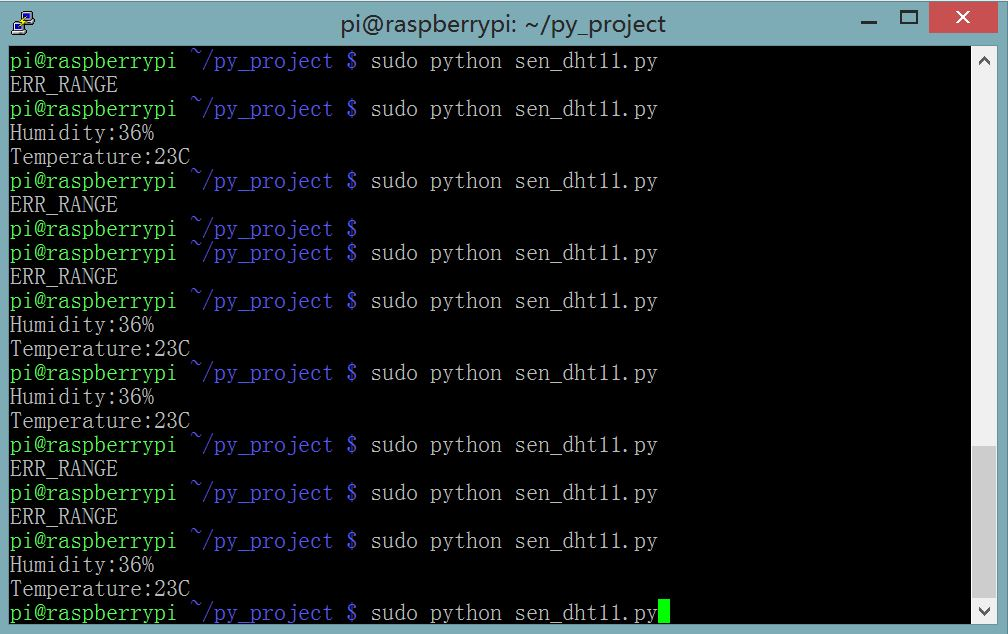We noticed that both examples has a chance to lose data. This is because Raspberry Pi is not running at a real-time system, the delay in the program is not accurate enough, and sometimes that leads to a failure of transmission. Because C is a lower level language, it controls the GPIO pin in a more direct way, so the example in C has less chance to fail.
As a work around, we can run the program for several times can pick the correct result.  This should also be possible to be done programmatically.